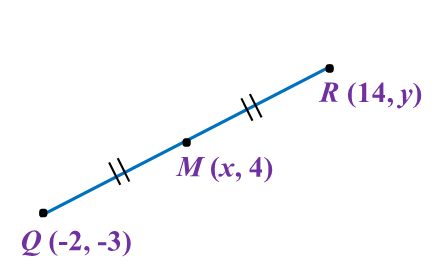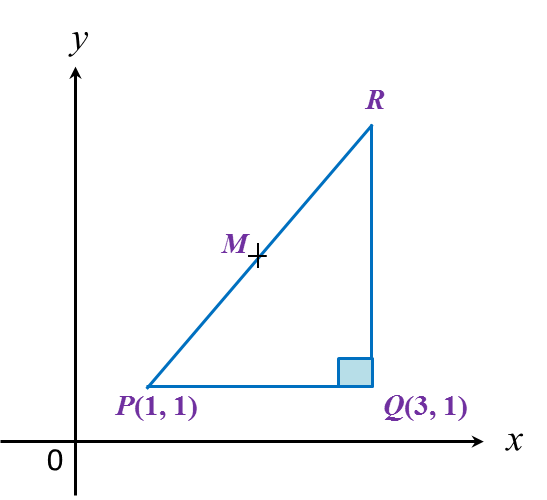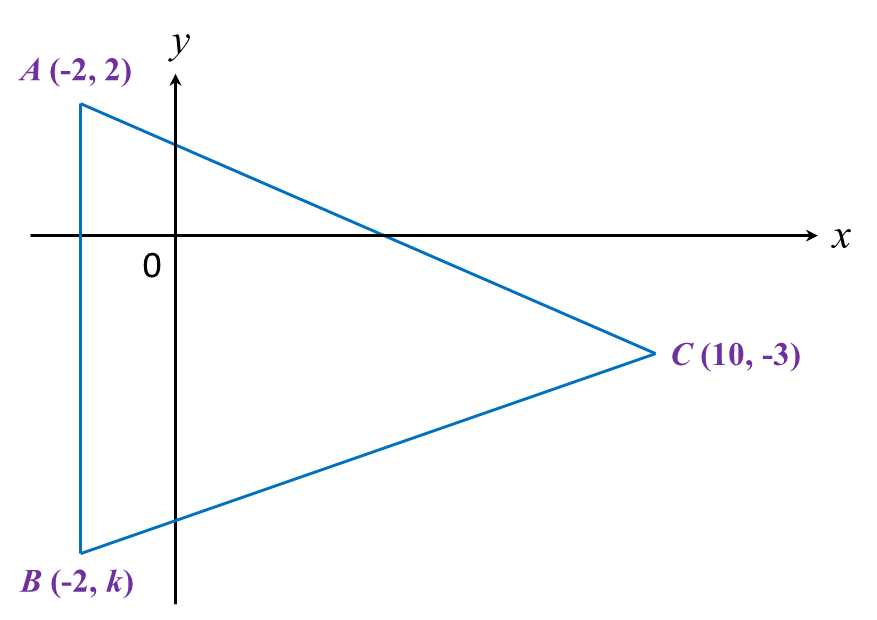# 8.2.2 Coordinates, PT3 Focus Practice

Question 6:
The point M (x, 4), is the midpoint of the line joining straight line Q (-2, -3) and R (14, y).The value of x and y are

Solution:

$\begin{array}{l}x=\frac{-2+14}{2}\\ x=\frac{12}{2}\\ x=6\\ \\ 4=\frac{-3+y}{2}\\ 8=-3+y\\ y=11\end{array}$

Question 7:
In diagram below, PQR is a right-angled triangle. The sides QR and PQ are parallel to the y-axis and the x-axis respectively. The length of QR = 6 units.Given that M is the midpoint of PR, then the coordinates of M are

Solution:
x-coordinate of R = 3
y-coordinate of R = 1 + 6 = 7
R = (3, 7)

Question 8:
Given points (–2, 8) and (10, 8), find the length of PQ.

Solution:

Question 9:
In diagram below, ABC is an isosceles triangle.Find
(a) the value of k,
(b) the length of BC.

Solution:

Question 10:
Diagram below shows a rhombus PQRS drawn on a Cartesian plane. PS is parallel to x-axis.Given the perimeter of PQRS is 40 units, find the coordinates of point R.

Solution:

# 8.2.3 Coordinates, PT3 Focus Practice

Question 11:
Diagram below shows the route of a Negaraku Run.The route of male participants is PQUT while the route for female participants is QRST. QR is parallel to UT whereas UQ is parallel to SR.
(a) Given the distance between point Q and point R is 9 km, state the coordinates of point R.
(b) It is given that the male participants start the run at point P and the female participants at point Q.
Find the difference of distance, in km, between the male and female participants.

Solution:
(a)
R = (9, 1)

(b)
Route of male participants
= PQ + QU + UT
= 10 + 7 + 7
= 24 km

Route of female participants
= QR + RS + ST
= 9 + 7 + 2
= 18 km

Difference in distance
= 24 – 18
= 6 km

Question 12:
Diagram below shows a parking lot in the shape of trapezium, RSTU. Two coordinates from the trapezium vertices are R(–30, –4) and S(20, –4).(a) Given the distance between vertex S and vertex T is 22 units.
State the coordinates of vertex T.
(b) Given the area of the parking lot is 946 unit2, find the coordinates of U.

Solution:
(a)
y-coordinates of T = –4 + 22 = 18
T = (20, 18)

(b)

# 8.2.1 Coordinates, PT3 Focus Practice

8.2.1 Coordinates, PT3 Focus Practice

Question 1
:
In diagram below, Q is the midpoint of the straight line PR.
The value of is

Solution
:
$\begin{array}{l}\frac{2+m}{2}=5\\ 2+m=10\\ \text{}m=8\end{array}$

Question 2:
In diagram below, P and Q are points on a Cartesian plane.

If M is the midpoint of PQ, then the coordinates of M are

Solution:

Question 3:
Find the distance between (–4, 6) and (20, –1).

Solution
:

Question 4:
Diagram shows a straight line PQ on a Cartesian plane.

Calculate the length, in unit, of PQ.

Solution
:
PS = 15 – 3 = 12 units
SQ = 8 – 3 = 5 units
By Pythagoras’ theorem,
PQ= PS2 + SQ2
= 122+ 52
PQ = √169
= 13 units

Question 5:
The diagram shows an isosceles triangle STU.

Given that ST = 5 units, the coordinates of point are

Solution
:

For an isosceles triangle STU, M is the midpoint of straight line TU.
Point M = (1, 0)

MT = 4 – 1 = 3 units
By Pythagoras’ theorem,
SM= ST2MT2
= 52 – 32
= 25 – 9
= 16
SM = √16
= 4
Therefore, point S = (1, 4).

# 8.1 Coordinates

8.1 Coordinates

8.1.1 Coordinates
1. The Cartesian coordinate system is a number plane with a horizontal line (x-axis) drawn at right angles to a vertical line (y-axis), intersecting at a point called origin.
2. It is used to locate the position of a point in reference to the x-axis and y-axis.

3. The coordinate of any point are written as an ordered pair (x, y).  The first number is the x-coordinate and the second number is the y-coordinate of the point.

Example:

The coordinates of points A and B are (3, 4) and (–5, –2) respectively.
This means that point A is located 3 units from they-axis and 4 units from the x-axis, whereas point B is located 5 units on the left from the y-axis and 2 units from the x-axis.

4. The coordinate of the origin O is (0, 0).

8.1.2 Scales for the Coordinate Axes
1. The scale for an axis is the number of units represented by a specific length along the axes.

2.
The scale on a coordinate is usually written in the form of a ratio.
Example:
A scale of 1 : 2 means one unit on the graph represents 2 units of the actual length.

3.
Both coordinate axes on the Cartesian plane may have
(a) the same scales, or
(b) different scales.
Example:

1 unit on the x-axis represents 2 units.
1 unit on the y-axis represents 1 unit.
Therefore the scale for x-axis is 1 : 2 and the scale for y-axis is 1 : 1.
Coordinates of:
(4, 3) and Q (10, 5).

8.1.3 Distance between Two Points
1. Finding the distance between two points on a Cartesian plane is the same as finding the length of the straight line joining them.

2. The distance between two points can be calculated by using Pythagoras’ theorem.
Example:
AB = 2 – (–4) = 2 + 4 = 6 units
BC = 5 – (–3) = 5 + 3 = 8 units
By Pythagoras’ theorem,
AC= AB2 + AC2
= 62 + 82
AC = √100
= 10 units
3. Distance is always a positive value.

8.1.4 Midpoint
The midpoint of a straight line joining two points is the middle point that divides the straight line into two equal halves.
$\text{Midpoint,}M=\left(\frac{{x}_{1}+{x}_{2}}{2},\frac{{y}_{1}+{y}_{2}}{2}\right)$

Example
:
The coordinate of the midpoint of (7, –5) and (–3, 11) are
$\begin{array}{l}\left(\frac{7+\left(-3\right)}{2},\frac{-5+11}{2}\right)\\ =\left(\frac{4}{2},\frac{6}{2}\right)\\ =\left(2,3\right)\end{array}$